#### Default Output for Maximum Likelihood

##### Optimization Information

The Optimization Information table displays basic information about the optimization setup to determine the maximum likelihood estimates, such as the optimization technique, the parameters that participate in the optimization, and the number of threads used for the calculations. This table is not produced during model selection—that is, if the KMAX= option is specified in the MODEL statement.

##### Iteration History

The Iteration History table displays for each iteration of the optimization the number of function evaluations (including gradient and Hessian evaluations), the value of the objective function, the change in the objective function from the previous iteration, and the absolute value of the largest (projected) gradient element. The objective function used in the optimization in the FMM procedure is the negative of the mixture log likelihood; consequently, PROC FMM performs a minimization. This table is not produced if the KMAX= option is specified in the MODEL statement.

##### Convergence Status

The convergence status table is a small ODS table that follows the Iteration History table in the default output. In the listing, it appears as a message that identifies whether the optimization succeeded and which convergence criterion was met. If the optimization fails, the message indicates the reason for the failure. If you save the Convergence Status table to an output data set, a numeric Status variable is added that allows you to assess convergence programmatically. The values of the Status variable encode the following:

0

Convergence was achieved or an optimization was not performed (because of TECHNIQUE=NONE).

1

The objective function could not be improved.

2

Convergence was not achieved because of a user interrupt or because a limit was exceeded, such as the maximum number of iterations or the maximum number of function evaluations. To modify these limits, see the MAXITER=, MAXFUNC=, and MAXTIME= options in the PROC FMM statement.

3

Optimization failed to converge because function or derivative evaluations failed at the starting values or during the iterations or because a feasible point that satisfies the parameter constraints could not be found in the parameter space.

##### Fit Statistics

The Fit Statistics table displays a variety of fit measures based on the mixture log likelihood in addition to the Pearson statistic. All statistics are presented in smaller is better form. If you are fitting a single-component normal, gamma, or inverse gaussian model, the table also contains the unscaled Pearson statistic. If you are fitting a mixture model or the model has been fitted under restrictions, the table also contains the number of effective components and the number of effective parameters.

The calculation of the information criteria uses the following formulas, where p denotes the number of effective parameters, n denotes the number of observations used (or the sum of the frequencies used if a FREQ statement is present), and l is the log likelihood of the mixture evaluated at the converged estimates: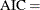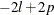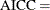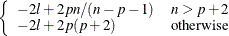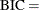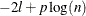The Pearson statistic is computed simply as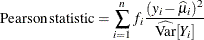where n denotes the number of observations used in the analysis,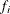is the frequency associated with the ith observation (or 1 if no frequency is specified),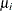is the mean of the mixture, and the denominator is the variance of the ith observation in the mixture. Note that the mean and variance in this expression are not those of the component distributions, but the mean and variance of the mixture: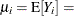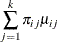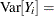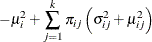where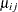and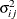are the mean and variance, respectively, for observation i in the jth component distribution and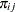is the mixing probability for observation i in component j.

The unscaled Pearson statistic is computed with the same expression as the Pearson statistic with n,, andas previously defined, but the scale parameteris set to 1 in the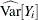expression.

The number of effective components and the number of effective parameters are determined by examining the converged solution for the parameters that are associated with model effects and the mixing probabilities. For example, if a component has an estimated mixing probability of zero, the values of its parameter estimates are immaterial. You might argue that all parameters should be counted towards the penalty in the information criteria. But a component with zero mixing probability in a k-component model effectively reduces the model to a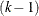-component model. A situation of an overfit model, for which a parameter penalty needs to be taken when calculating the information criteria, is a different situation; here the mixing probability might be small, possibly close to zero.

##### Parameter Estimates

The parameter estimates, their estimated (asymptotic) standard errors, and p-values for the hypothesis that the parameter is zero are presented in the Parameter Estimates table. A separate table is produced for each MODEL statement, and the components that are associated with a MODEL statement are identified with an overall component count variable that counts across MODEL statements. If you assign a label to a model with the LABEL= option in the MODEL statement, the label appears in the title of the Parameter Estimates table. Otherwise, the internal label generated by the FMM procedure is used.

If the MODEL statement does not contain effects and the link function is not the identity, the inversely linked estimate is also displayed in the table. For many distributions, the inverse linked estimate is the estimated mean on the data scale. For example, in a binomial or binary model, it represents the estimated probability of an event. For some distributions (for example, the Weibull distribution), the inverse linked estimate is not the component distribution mean.

If you request confidence intervals with the CL or ALPHA= option in the MODEL statement, confidence limits are produced for the estimate on the linear scale. If the inverse linked estimate is displayed, confidence intervals for that estimate are also produced by inversely linking the confidence bounds on the linear scale.

##### Mixing Probabilities

If you fit a model with more than one component, the table of mixing probabilities is produced. If there are no effects in the PROBMODEL statement or if there is no PROBMODEL statement, the parameters are reported on the linear scale and as mixing probabilities. If model effects are present, only the linear parameters (on the scale of the logit, generalized logit, probit, and so on) are displayed.